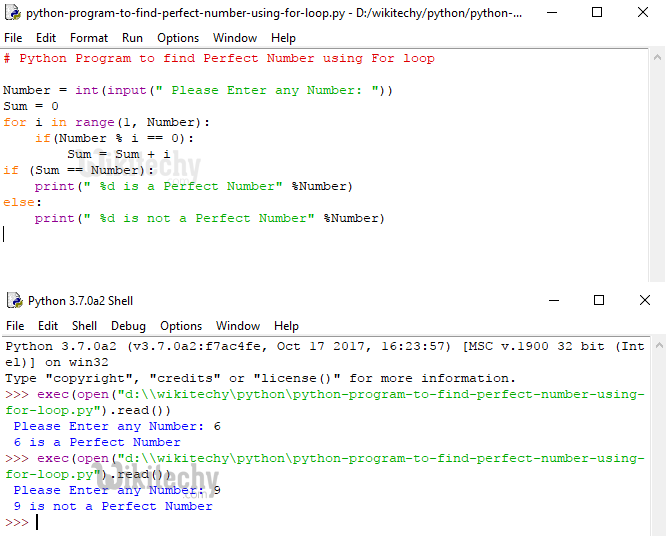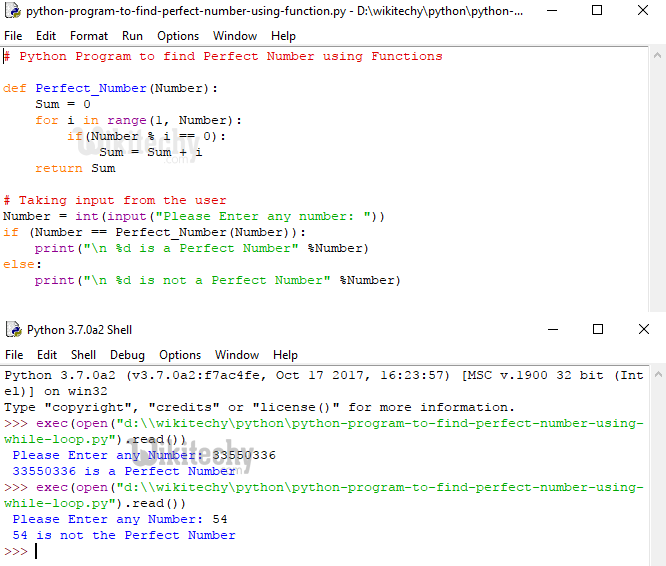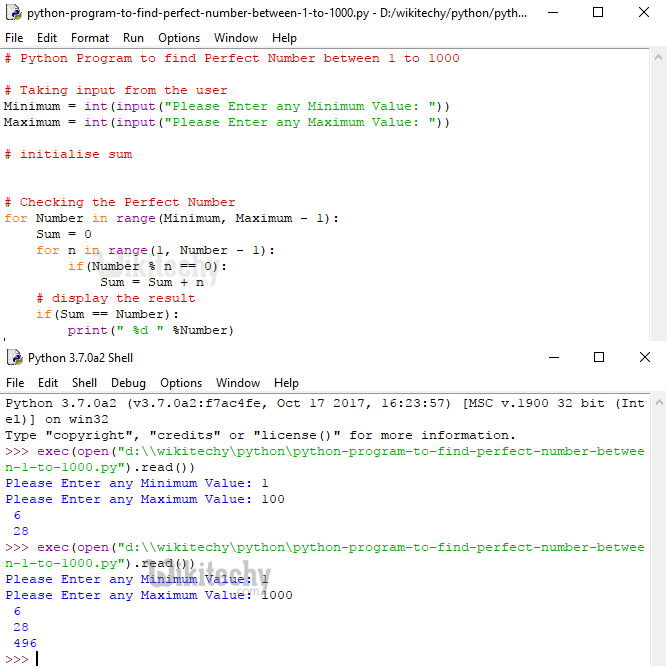# python tutorial - Python Program to find Perfect Number - learn python - python programming

## Perfect Number

• Any number can be perfect number, if the sum of its positive divisors excluding the number itself is equal to that number.
• For example, 6 is a perfect number because 6 is divisible by 1, 2, 3 and 6.
• So, the sum of these values are: 1+2+3 = 6 (Remember, we have to exclude the number itself.
• That’s why we haven’t added 6 here). Some of the perfect numbers are 6, 28, 496, 8128 and 33550336 so on.
• How to write a Python program to find Perfect Number using For Loop, While Loop, and Functions.Learn Python - Python tutorial - Python Program to find Perfect Number - Python examples - Python programs

## Python Program to find Perfect Number using For loop

• This Python program allows the user to enter any number.
• Using this number it will calculate whether the number is Perfect number or not using the Python For Loop.

## Analysis

• Following statement will ask the user to enter any number and stores the user entered value in variable Number
• Next line, We declared integer variable Sum = 0.
• Next, we used the for loop with range() and we are not going to explain the for loop here.
• Inside the For loop we placed Python If Statement to test the condition
• If statement inside the for loop will check whether the Number is Perfectly divisible by i value or not.
• f the Number is perfectly divisible by i then i value will be added to the Sum.

## OutputLearn Python - Python tutorial - Python Program to find Perfect Number using For loop - Python examples - Python programs

• From the above Screenshot, User Entered value is: Number = 6

## First Iteration

For the first Iteration, Number = 6, Sum = 0 and i = 1 If (Number % i == 0) 6 % 1 == 0 If statement is succeeded here So, Sum = Sum + i Sum = 0 +1 = 1

## Second Iteration

For the second Iteration the values of both Sum and i has been changed as: Sum = 1 and i = 2 If (Number % i == 0) 6 % 2 == 0 If statement is succeeded here So, Sum = Sum + i Sum = 1 + 2 = 3

## Third Iteration

For the third Iteration the values of both Sum and i has been changed as: Sum = 3 and i = 3 If (Number % i == 0) 6 % 3 == 0 If statement is succeeded here So, Sum = Sum + i Sum = 3 + 3 = 6 For the fourth and fifth iterations condition inside the if condition will fail 6 % 4 == 0 (FALSE) 6 % 5 == 0 (FALSE)

• So, compiler will terminate the for loop.
• In the next line we have If statement to check whether the value inside the Sum variable is exactly equal to given Number or Not.
• If the condition (Sum == Number) is TRUE then below print statement will be executed
• If the condition (Sum == Number) is FALSE then below print statement will be executed
• For this example (Sum == Number) is True. So, given Number is Perfect Number

## Python Program to find Perfect Number using While Loop

• This program allows the user to enter any number. Using this number it will calculate whether the user input is Perfect number or not using Python While loop.

## Sample Code

• We haven’t done anything special in this example.
• We just replaced the For loop with While Loop and if you find difficult to understand the While loop functionality

## OutputLearn Python - Python tutorial - Python Program to find Perfect Number using While Loop - Python examples - Python programs

## Python Program to find Perfect Number using Function

• This program allows the user to enter any number.
• Using this number it will calculate whether the number is Perfect number or not using the Functions.

## Sample Code

• If you observe the above code, We haven’t done anything special in this example.
• We just defined the new user defined function and added the code we mentioned in For loop example.

## OutputLearn Python - Python tutorial - Python Program to find Perfect Number using Function - Python examples - Python programs

## Python Program to Find Perfect Number between 1 to 1000

• This program allows the user to enter minimum and maximum values. This program will find the Perfect Number between the Minimum and Maximum values.

## OutputLearn Python - Python tutorial - Python Program to Find Perfect Number between 1 to 1000 - examples - programs

## Analysis

• This For Loop helps compiler to iterate between Minimum and Maximum Variables, iteration starts at the Minimum and then it will not exceed Maximum variable.